### Jonathan M. Hoekstra, Krista K. Bartz, Mary H. Ruckelshaus, Jennifer M. Moslemi, and Tamara K. Harms. 2007. Quantitative threat analysis for management of an imperiled species: Chinook salmon (Oncorhynchus tshawytscha). Ecological Applications 17:2061–2073.

Appendix A. Supplemental methods.

Following Burnham and Anderson (2002), model-averaged estimates of effects (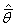) for each confidence set were calculated as: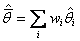where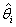was the maximum likelihood estimate of an effect from model i (or zero if the associated effect was excluded from the model variation) and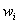was the Akaike weight of model i. Model-averaged variance estimates (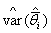) also were calculated:wherewas the Akaike weight of model i,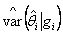was the bootstrap-derived estimate of variance about the maximum-likelihood estimate offrom model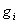, andwas the model-averaged estimate of the parameter of interest. If an effect was excluded from model,andwere fixed to zero. This formulation for estimating model-averaged variance included a component reflecting model selection uncertainty and thus was not conditioned on any single model in the confidence set.

[Back to A017-081]